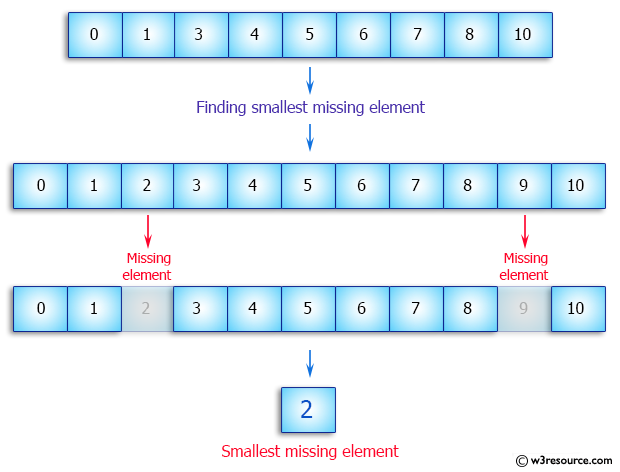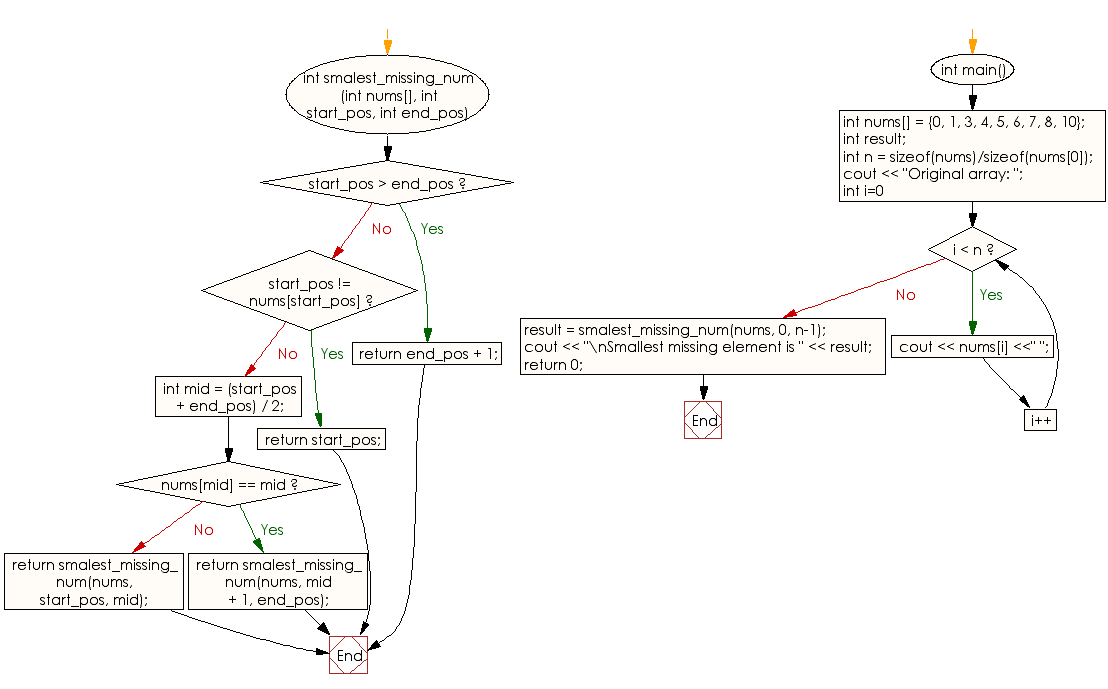﻿ C++ Exercises: Find the smallest element missing in a sorted array - w3resourceC++ Exercises: Find the smallest element missing in a sorted array

C++ Array: Exercise-10 with Solution

Write a C++ program to find the smallest element missing in a sorted array.

Pictorial Presentation:Sample Solution:

C++ Code :

#include<iostream>
#include<algorithm>
using namespace std;

int smalest_missing_num(int nums[], int start_pos, int end_pos)
{
if (start_pos  > end_pos)
return end_pos + 1;

if (start_pos != nums[start_pos])
return start_pos;

int mid = (start_pos + end_pos) / 2;

if (nums[mid] == mid)
return smalest_missing_num(nums, mid + 1, end_pos);

return smalest_missing_num(nums, start_pos, mid);
}

int main()
{
int nums[] = {0, 1, 3, 4, 5, 6, 7, 8, 10};
int result;
int n = sizeof(nums)/sizeof(nums);
cout << "Original array: ";
for (int i=0; i < n; i++)
cout << nums[i] <<" ";
result = smalest_missing_num(nums, 0, n-1);
cout << "\nSmallest missing element is " << result;
return 0;
}

Sample Output:

Original array: 0 1 3 4 5 6 7 8 10
Smallest missing element is 2

Flowchart:C++ Code Editor: